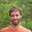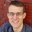Question-and-Answer Resource for the Building Energy Modeling Community
Get started with the Help page

Output to calculate the heating and cooling loadWhich is the correct output to calculate the thermal/cooling energy request (kWh) and rate (W) to reach the setpoint temperature? I found many different outputs giving slight differences when calculating the energy consumptions. At the moment I'm considering the output "Zone predicted Sensible Load to Setpoint Heat Transfer Rate" (W) ; is it the correct one? It has positive values for heating load and negative ones for cooling.

Is it better, instead, to consider the "Zone Air System sensible heating/cooling energy/rate" or something else?

Thanks for the help.

edit retag close merge delete

Sort by » oldest newest most votedWhile the common answer is to use the Zone Predicted Sensible Load to Setpoint Heat Transfer Rate variable, I would not recommend it. It is true that this variable will often give a good approximate answer, but it is not guaranteed.

The reason for this is that the output variable calculates the heating/cooling energy to take the zone temperature (after applying heat transfer from surfaces, internal gains, etc. to the starting zone temperature from the previous timestep) to setpoint. If the starting zone temperature from the previous timestep was at setpoint, this calculation is correct. But if the starting zone temperature from the previous timestep was not perfectly maintained at setpoint (e.g., because the zone is under conditioned due to a HVAC system with insufficient capacity), the calculation ends up larger than the actual load. To the extent that the setpoint is not perfectly maintained by the HVAC system, the calculation ends up further off.

Instead, I recommend using ZoneHVAC:IdealLoadsAirSystem object. The object can perfectly maintain setpoint. When using this object, you likely want to disable outdoor air, dehumificiation, and humidification. Then look at its Zone Air System Sensible Heating Energy and Zone Air System Sensible Cooling Energy outputs.

moremore